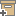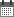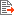ניהול
קהילה:
אסיף מאגר המחקר החקלאי
Estimating the Soil Water Dependence of the Electrical Conductivity Soil Solution/Electrical Conductivity Bulk Soil RatioBack to searchPrevious item
Next item
Year:
1982
Authors :
נדלר, אריה
;
.
Volume :
46
Co-Authors:
Facilitators :
From page:
722
To page:
726
(
Total pages:
5
)
Abstract:

A procedure is developed to calculate the ratio between electrical conductivity (EC) solution (ECw) and EC of bulk soil (ECa) as a function of soil water content (θ). This ratio, (F), changing nonlinearly with θ, is used to calculate soil solution salinity from measurements of bulk soil electrical conductivity. Obtaining F‐θ relationships for intermediate and low soil moisture levels by an accurate theoretical model is impractical due to the complicated interdependence of several solid‐ and liquid‐phase parameters. Therefore an empirical approach was adopted to obtain the complete F‐θ relationship by superimposing the complete suction‐water retention relationship on two F‐θ measurements at the same θ values. The experimentally determined F‐θ curve overlaps the theoretically calculated F‐θ relationship at high soil water contents and the empirically obtained F‐θ relationship at low soil water content. Examples are given for several soil types.

Note:
Related Files :
FOUR-ELECTRODE PROBE
Pore-size distribution
soil
SOIL ELECTRICAL CONDUCTIVITY
Soil salinity
water
עוד תגיות
תוכן קשור
More details
DOI :
https://doi.org/10.2136/sssaj1982.03615995004600040011x
Article number:
0
Affiliations:
Database:
Publication Type:
מאמר
;
.
Language:
אנגלית
Editors' remarks:
ID:
50591
Last updated date:
02/03/2022 17:27
Creation date:
30/09/2020 08:06Scientific Publication
Estimating the Soil Water Dependence of the Electrical Conductivity Soil Solution/Electrical Conductivity Bulk Soil Ratio
46
Estimating the Soil Water Dependence of the Electrical Conductivity Soil Solution/Electrical Conductivity Bulk Soil Ratio

A procedure is developed to calculate the ratio between electrical conductivity (EC) solution (ECw) and EC of bulk soil (ECa) as a function of soil water content (θ). This ratio, (F), changing nonlinearly with θ, is used to calculate soil solution salinity from measurements of bulk soil electrical conductivity. Obtaining F‐θ relationships for intermediate and low soil moisture levels by an accurate theoretical model is impractical due to the complicated interdependence of several solid‐ and liquid‐phase parameters. Therefore an empirical approach was adopted to obtain the complete F‐θ relationship by superimposing the complete suction‐water retention relationship on two F‐θ measurements at the same θ values. The experimentally determined F‐θ curve overlaps the theoretically calculated F‐θ relationship at high soil water contents and the empirically obtained F‐θ relationship at low soil water content. Examples are given for several soil types.

Scientific Publication
You may also be interested in